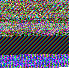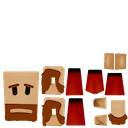#### Howdy, Stranger!

It looks like you're new here. If you want to get involved, click one of these buttons!

# Blender model exporter for Codea

edited February 2013 Posts: 196

Hello all,

I felt that it would be really cool to have more complex looking 3D scenes running in Codea, and working with blender is pretty easy so well...

edit: I've rewritten the exporter a while ago, but updates to this post have been lacking. This is now a fully functional version

You can find the latest exporter on my website here

It will take a mesh and export it to an image, like this one:This really makes it much more usable to use and share than having huge text files in your codea project.
Unfortunately, I can only use RGB to encode the data, using the alpha channel makes it unusable in Codea due to the way textures are loaded onto a surface). this means the output file is slightly larger

Vertices, vertices colors, normals and UVs (texture coordinates) are exported.

Simple textured monkey example:Anyway, it's simple enough to use, create your pretty scene, hit export and you get an image ready to import into blender.

Usage is pretty simple (this is normally part of a class used in my upcoming project, but I cleaned it up to be functions).
Here are the images used in the following example: http://ezakirth.free.fr/files/and``````--# Main
function setup()
mdl = Model("Dropbox:model", "Dropbox:texture")

end
ang = 0
end

function draw()
background(0)

perspective(60)
local x = math.sin(ang)*5
local y = math.cos(ang)*5
local z = math.cos(ang)*5
camera(x, y, z, 0, 0, 0, 0, 0, 1)

mdl:draw()

ang = ang + (math.pi/180)
end

--# Model
function Model(mdl, texture)
local width, height = spriteSize(mdl)

local verts = {}
local normals = {}
local colors = {}
local coords = {}

local a1, b1, c1, d1, a2, b2, c2, d2, a3, b3, c3, d3, x, y, z, X, Y, n, size
local strToChar = string.char
local ins = table.insert

cnt = 0
X = 1
Y = height

n = 1
a1, b1, c1 = img:get(X,Y)
X, Y = nextPixel(X, Y, width)
d1 = img:get(X,Y)
X, Y = nextPixel(X, Y, width)
size = bytesToFloat(strToChar(a1, b1, c1, d1))

cnt = 0
-- vertices
while n<=size do
a1, b1, c1 = img:get(X,Y)
X, Y = nextPixel(X, Y, width)

d1, a2, b2 = img:get(X,Y)
X, Y = nextPixel(X, Y, width)

c2, d2, a3 = img:get(X,Y)
X, Y = nextPixel(X, Y, width)

b3, c3, d3 = img:get(X,Y)
X, Y = nextPixel(X, Y, width)

x = bytesToFloat(strToChar(a1, b1, c1, d1))
y = bytesToFloat(strToChar(a2, b2, c2, d2))
z = bytesToFloat(strToChar(a3, b3, c3, d3))

ins(verts, vec3(x, y, z))
n = n + 1
end
print(cnt.." for "..size.." vertices")
cnt = 0
-- normals
n = 1
while n<=size do
a1, b1, c1 = img:get(X,Y)
X, Y = nextPixel(X, Y, width)

d1, a2, b2 = img:get(X,Y)
X, Y = nextPixel(X, Y, width)

c2, d2, a3 = img:get(X,Y)
X, Y = nextPixel(X, Y, width)

b3, c3, d3 = img:get(X,Y)
X, Y = nextPixel(X, Y, width)

x = bytesToFloat(strToChar(a1, b1, c1, d1))
y = bytesToFloat(strToChar(a2, b2, c2, d2))
z = bytesToFloat(strToChar(a3, b3, c3, d3))

ins(normals, vec3(x, y, z))
n = n + 1
end
print(cnt.." for "..size.." normals")
cnt = 0
-- colors
n = 1
while n<=size do
a1, b1, c1 = img:get(X,Y)
X, Y = nextPixel(X, Y, width)

d1, a2, b2 = img:get(X,Y)
X, Y = nextPixel(X, Y, width)

c2, d2, a3 = img:get(X,Y)
X, Y = nextPixel(X, Y, width)

b3, c3, d3 = img:get(X,Y)
X, Y = nextPixel(X, Y, width)

x = bytesToFloat(strToChar(a1, b1, c1, d1))*255
y = bytesToFloat(strToChar(a2, b2, c2, d2))*255
z = bytesToFloat(strToChar(a3, b3, c3, d3))*255

ins(colors, color(x, y, z, 255))
n = n + 1
end
print(cnt.." for "..size.." colors")
cnt = 0

-- texture coordinates
if texture then
n = 1
while n<=size do
a1, b1, c1 = img:get(X,Y)
X, Y = nextPixel(X, Y, width)

d1, a2, b2 = img:get(X,Y)
X, Y = nextPixel(X, Y, width)

c2, d2, a3 = img:get(X,Y)
X, Y = nextPixel(X, Y, width)

x = bytesToFloat(strToChar(a1, b1, c1, d1))
y = bytesToFloat(strToChar(a2, b2, c2, d2))

ins(coords, vec2(x,y))
n = n + 1
end
end

local total = 3*(size*4)+(size*3)+2
print(cnt.." for "..size.." texture coords")
print("total:"..total.." pixels, for a model of "..(size/3).." polygons")

local min = math.ceil(math.sqrt(total))
print("min img size:"..min.."x"..min)
cnt = 0

local data = mesh()
data.vertices = verts
data.colors = colors
data.normals = normals

if texture then
data.texture = texture
data.texCoords = coords
end
else
end
end

verts = nil
coords = nil
colors = nil
normals = nil
img = nil

return data
end

-- Converts a string of 4 bytes to a float
function bytesToFloat(x)
local byte = string.byte
local ldexp = math.ldexp
local sign = 1
local mantissa = byte(x, 3) % 128
for i = 2, 1, -1 do
mantissa = mantissa * 256 + byte(x, i)
end
if byte(x, 4) > 127 then
sign = -1
end
local exponent = (byte(x, 4) % 128) * 2 + math.floor(byte(x, 3) / 128)
if exponent == 0 then
return 0
end
mantissa = (ldexp(mantissa, -23) + 1) * sign
return ldexp(mantissa, exponent - 127)
end

-- Goes to next available pixel
function nextPixel(x, y, max)
cnt = cnt + 1
x = x + 1
if x>max then
x = 1
y = y - 1
end
return x, y
end
``````

Cheers,

Xavier

Tagged:

• Posts: 1,557

Very cool. I imagine the classes are going to be huge...

• Posts: 196

@Bortels

Nah, the Monkey file for example is only 23658 lines of code and weighs 900kb :P
It has a super high polygon count though, I could have had a much lower resolution mesh while not losing much visual quality.

Xavier

• Posts: 115

Very interesting indeed. Thanks for working on an exporter to use in Codea.

I see I have only Blender 2.49 and a 2.56 beta installed. Time for an update. I never got very comfortable with Blender but the other modellers I use can export to Blender.

Looking forward to where this may lead us, graphically.

• Posts: 2,820

YES! THANK YOU! This is awsome!

• Posts: 398

Excellent stuff! Im working on a similar one for Maya (albeit for simple polygonal entities) as well as a simple .obj exporter (via Python) - will keep you posted ;-)

• Posts: 196

Sup sup,

Really sorry, I have been very busy and haven't made progress on thisUntil then, here is the last source for the exporter: http://pastebin.com/im8asb4U

I'll finish this one soon

Xavier

• Posts: 196

updated the original post. note that you can now only export one object at a time, and no longer a scene like in the previous version

• Posts: 9

Xavier just tried to load the .py file in blender 2.65, I edited the version to 2.6.5 and it now shows in the addon view but it refuses to be enabled when clicking on the toggle.
I loaded the .py script in a text viewer in blender and ran it and it threw errors on the "from PIL import Image, ImageDraw", No modual named PIL.
Does this mean the PIL python libary has to be seperatly be installed?

• Posts: 196

. @nozzy oh jeez yeah, i'm using the PIL (python image library) to handle the .png image generation...I'm sorry for the confusion, this is something I use personally, wasn't really made to be shared.
Here is a link to the PIL : http://www.pythonware.com/products/pil/

Let me know if you have any issues

• Posts: 9

Ok, great i'll try and get PIL in, i remember having problems with Win7 64bit and installing PIL before. Thanks Xavier.

• Posts: 437

This is amazing, does it has animation support too?

• Posts: 1

As a blender head myself, this is very cool, never expected to see a crossover of any kind when I started using codea.

• Posts: 2

Tried PIL myself but still can't 'activate' the addon in Blender 2.57, 2.59 or 2.68 ?
I installed Python (32 bit & 64 bit) and PIL. I Am using Win 7 ultimate 64bit. Any tips please?

Thanks• Posts: 3,297

Since it is really a python problem, maybe ask the pythonista community? http://omz-software.com/pythonista/forums/

• Posts: 2

Will give it a shot! Thanks Jmv38